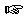### Section 9

Problem 1:

Develop the function between?, which consumes three numbers and produces `true` if the last is between the first two, otherwise it returns `false`.

Use between? to develop the function three-between?, which consumes two numbers and a list of three numbers and determines if all three numbers in the list are between the first two.Solution

Problem 2:

Develop a function that consumes a list of booleans and determines whether one of them is `true`. Consider whether `empty` contains a `true` element.Solution

Problem 3:

Develop a function that consumes a list of numbers and computes the difference between the number of positive numbers in the list and the number of negative numbers in the list.Solution

Problem 4:

Develop the function string-append-n*, which consumes a non-empty list of strings and produces a single string resulting from appending all of the strings together. Use the built-in function string-append: `String String -> String`, which appends two strings.

Now develop the more general function string-append*, which consumes any list of strings and produces a single string as described above. This new function must handle empty in reasonable way.Solution

Problem 5:

Develop the function order-toy, which consumes a symbol representing a toy, a number representing how many of that particular toy you wish to have in stock, and a list of symbols representing an inventory of toys. It produces the number copies that need to be ordered. Consider using the how-many-symbols function from Exercise 9.5.2 as a helper.Solution

 Jamie Raymond Matthias Felleisen 01 december 2003